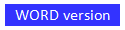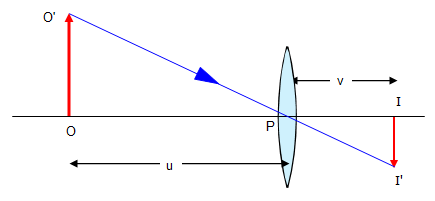# Magnification by a lens

Lenses give an image of the object. The size of the image compared with the object depends on the magnification of the lens.
The magnification of a lens is defined as:

Magnification = image height (II')/object height (OO')

In spite of what you might think magnifications don't always have to be bigger than one. For example in a camera and in your eyes the image is smaller than the object.However if we look at the diagram and consider the triangles OO'P and II'P we can see that the magnification is also equal to image distance/object distance or v/u.

For a convex lens with an object distance of 15 cm and an image distance of 7.5 cm the magnification would be 7.5/15 or +0.5, the image is real and half the size of the object.

For a convex lens with an object distance of 8 cm and an image distance of -24 cm the magnification is -24/8 or -3, the image is virtual and three times the size of the object.

Example problem
An object is placed 4cm from a convex lens of focal length 12 cm. Where is the image and what is the magnification?
u = 4cm    f = + 12cm
1/4 + 1/v = 1/12    so    v = - 6 cm.
Magnification = v/u = - 6/4 = - 1.5.

The image is virtual ( 6 cm behind the lens) and 1.5 times bigger than the object

© Keith Gibbs 2013